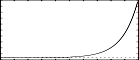## GEN51

GEN51 — This subroutine fills a table with a fully customized micro-tuning scale, in the manner of Csound opcodes cpstun, cpstuni and cpstmid.

## Description

This subroutine fills a table with a fully customized micro-tuning scale, in the manner of Csound opcodes cpstun, cpstuni et cpstmid.

## Syntax

`f # time size -51 numgrades interval basefreq basekey tuningRatio1 tuningRatio2  .... tuningRationN`

## Performance

The first four parameters (i.e. p5, p6, p7 and p8) define the following generation directives:

p6 (interval) -- the frequency range covered before repeating the grade ratios, for example 2 for one octave, 1.5 for a fifth etcetera

p7 (basefreq) -- the base frequency of the scale in cps

p8 (basekey) -- the integer index of the scale to which to assign basefreq unmodified

The other parameters define the ratios of the scale:

p9...pN (tuningRatio1...etc.) -- the tuning ratios of the scale

For example, for a standard 12-grade scale with the base-frequency of 261 cps assigned to the key-number 60, the corresponding f-statement in the score to generate the table should be:

```;             numgrades        basefreq           tuning-ratios  (eq.temp) .......
;                     interval         basekey
f1 0 64 -51     12       2      261      60        1   1.059463 1.12246 1.18920 ..etc...```

After the gen has been processed, the table f1 is filled with 64 different frequency values. The 60th element is filled with the frequency value of 261, and all other elements (preceding and subsequents) of the table are filled according to the tuning ratios

Another example with a 24-grade scale with a base frequency of 440 assigned to the key-number 48, and a repetition interval of 1.5:

```;            numgrades       basefreq             tuning-ratios .....
;                     interval         basekey
f1 0 64 -51     24      1.5     440      48         1   1.01  1.02  1.03  ..etc...```

## Examples

Here is an example of the GEN51 routine. It uses the files gen51.csd.

Example 1313. An example of the GEN51 routine.

```<CsoundSynthesizer>
<CsOptions>
; Select audio/midi flags here according to platform
-odac   -M0    ;;;realtime audio out and midi input
; For Non-realtime ouput leave only the line below:
; -o gen51.wav -W ;;; for file output any platform
</CsOptions>
<CsInstruments>
;example by Iain McCurdy
sr = 44100
ksmps = 32
nchnls = 2
0dbfs  = 1

giEqTmp12	ftgen	1,0,128,-51,12,2,cpsoct(8),60,1,2^(1/12),2^(2/12),2^(3/12),2^(4/12),2^(5/12),2^(6/12),2^(7/12),2^(8/12),2^(9/12),2^(10/12),2^(11/12),2^(12/12)
giEqTmp10	ftgen	2,0,128,-51,10,2,cpsoct(8),60,1,2^(1/10),2^(2/10),2^(3/10),2^(4/10),2^(5/10),2^(6/10),2^(7/10),2^(8/10),2^(9/10),2^(10/10)
giEqTmp24	ftgen	3,0,128,-51,24,2,cpsoct(8),60,1,2^(1/24),2^(2/24),2^(3/24),2^(4/24),2^(5/24),2^(6/24),2^(7/24),2^(8/24),2^(9/24),2^(10/24),2^(11/24), \ 2^(12/24),2^(13/24),2^(14/24),2^(15/24),2^(16/24),2^(17/24),2^(18/24),2^(19/24),2^(20/24),2^(21/24),2^(22/24),2^(23/24),2^(24/24)

instr	1	;midi input instrument
/*USE PITCH BEND TO MODULATE NOTE NUMBER UP OR DOWN ONE STEP - ACTUAL INTERVAL IT WILL MODULATE BY WILL BE DEPENDENT UPON THE GEN51 SCALE USED*/
;kbend	pchbend	0,2

/*ALTERNATIVELY IF USING VIRTUAL MIDI DEVICE OR A KEYBOARD WITH NO PITCH BEND WHEEL, USE CONTROLLERS 1 AND 2 TO MODULATE PITCH UP OR DOWN 1 STEP*/
kup	ctrl7	1, 1, 0, 1
kdown	ctrl7	1, 2, 0, -1
kbend	=	kup+kdown

inum	notnum
kcps	tablei	inum+kbend, giEqTmp24	;read cps values from GEN51, scale table using a combination of note played and pitch bend/controllers 1 and 2
a1	vco2	0.2, kcps, 4, 0.5
outs	a1, a1
endin

instr	2	;score input instrument

knum	line	p4, p3, p5		;gliss using a straight line bewteen p4 and p5 for the entire note duration
kcps	tablei	knum, giEqTmp24		;read cps values from GEN51 scale table
a1	vco2	0.2, kcps, 4, 0.5
outs	a1, a1
endin

</CsInstruments>
<CsScore>
f 0 3600

;instr 2. Score input. Gliss from step number p4 to step number p5
;p4 - starting note number
;p5 - ending note number
i 2 0 2 60    61
i 2 + 2 70    58
i 2 + 2 66    66.5
i 2 + 2 71.25 71
e
</CsScore>
</CsoundSynthesizer>
```

These are the diagrams of the waveforms of the GEN51 routines, as used in the example:f 1 0 128 -51 12 2 cpsoct(8) 60 1 2^(1/12) 2^(2/12) 2^(3/12) 2^(4/12) 2^(5/12) 2^(6/12) 2^(7/12) 2^(8/12) 2^(9/12) 2^(10/12) 2^(11/12) 2^(12/12)f 2 0 128 -51 10 2 cpsoct(8) 60 1 2^(1/10) 2^(2/10) 2^(3/10) 2^(4/10) 2^(5/10) 2^(6/10) 2^(7/10) 2^(8/10) 2^(9/10) 2^(10/10)f 3 0 128 -51 24 2 cpsoct(8) 60 1 2^(1/24) 2^(2/24) 2^(3/24) 2^(4/24) 2^(5/24) 2^(6/24) 2^(7/24) 2^(8/24) 2^(9/24) 2^(10/24) 2^(11/24) 2^(12/24) 2^(13/24) 2^(14/24) 2^(15/24) 2^(16/24) 2^(17/24) 2^(18/24) 2^(19/24) 2^(20/24) 2^(21/24) 2^(22/24) 2^(23/24) 2^(24/24)